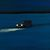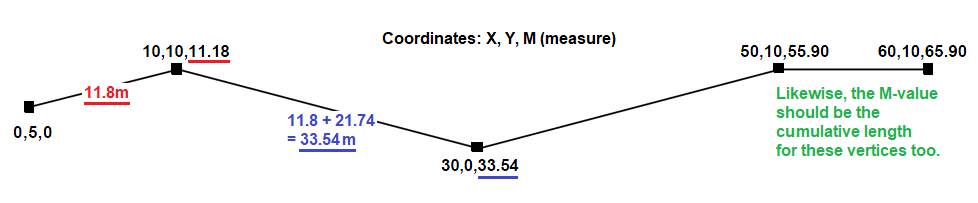# Arcade Code Review: Set polyline M-values to cumulative length of line

1086
12
03-11-2022 10:19 AM
Labels (1)by
Frequent Contributor II

I have an Arcade calculation attribute rule that sets polyline M-values to the cumulative length of the line.The script works as expected. But I'm still a novice, so I thought I'd ask: Can the script be improved?

- It works for both singlepart and multipart features.
- It densifies true curves (unavoidable, since Arcade pre-densifies true curves).
- The code below is out-of-date. I've posted an updated version in a reply...further down in this post.

```//var paths = [[[0, 5, null], [10, 10, null], [30, 0, null], [50, 10, null], [60, 10, null]]];  //JS

//Only do the calculation if the geometry was changed/edited.
if (!Equals(Geometry(\$feature), Geometry(\$originalfeature))) {
var geom = Dictionary(Text(Geometry(\$feature)));
var paths = geom['paths'];

//Alternatively, this could be done with the Arcade distance() function. I chose to do the math manually so that the script can be used elsewhere, such as VSCode (for debugging).
function pythagoras(x1, y1, x2, y2) {
return sqrt(pow(x2 - x1, 2) + pow(y2 - y1, 2));
}
for (var path_idx in paths) {
var oldX = paths, oldY = paths, length = 0;
for (var point_idx in paths[path_idx]) {
var newX = paths[path_idx][point_idx], newY = paths[path_idx][point_idx];
length += pythagoras(oldX, oldY, newX, newY);
paths[path_idx][point_idx] = length;
oldX = newX;
oldY = newY;
}
}
console(paths);
return { "result": { "geometry": Polyline(geom) } };

//Output: [[[0,5,0],[10,10,11.180339887498949],[30,0,33.54101966249685],[50,10,55.90169943749475],[60,10,65.90169943749476]]]
}  ```

(The script was adapted from this GitHub sample: Set Ms to Index.)

Thanks!

Tags (1)
12 RepliesbyEsri Frequent Contributor

Looks pretty good, minor tweaks and comments

`//Only do the calculation if the geometry was changed/edited.// I like to exit early when condition is not met, makes the code a little more readableif (Equals(Geometry(\$feature), Geometry(\$originalfeature))) {     return}var geom = Dictionary(Text(Geometry(\$feature))); var paths = geom['paths']; //Alternatively, this could be done with the Arcade distance() function. I chose to do the math manually so that the script can be used elsewhere, such as VSCode (for debugging).function pythagoras(x1, y1, x2, y2) {    return sqrt(pow(x2 - x1, 2) + pow(y2 - y1, 2));}// Easier to read when on multi lines and moved out of the loop, it was being reset on each part, not sure that is what you wantedvar oldX = paths;var oldY = paths;// dont use length, that is a reserved function namevar line_length = 0;for (var path_idx in paths) {    for (var point_idx in paths[path_idx]) {        var newX = paths[path_idx][point_idx];        var newY = paths[path_idx][point_idx];        line_length += pythagoras(oldX, oldY, newX, newY);        // can use a negative slice, will work when there is no Z        paths[path_idx][point_idx][-1] = line_length;        oldX = newX;        oldY = newY;    }}  console(paths);return { "result": { "geometry": Polyline(geom) } };//Output: [[[0,5,0],[10,10,11.180339887498949],[30,0,33.54101966249685],[50,10,55.90169943749475],[60,10,65.90169943749476]]]}  //Generic JS version: https://jsfiddle.net/5m1c69g3/`by
Frequent Contributor II

Thanks very much Mike. That really helps.by
Frequent Contributor II

``````//Script title: Set polyline M-values to cumulative length of line

var geom = Dictionary(Text(Geometry(\$feature)));
var paths = geom['paths'];

//Using the Equals() function doesn't work as expected. See: Arcade: Editing M-values not treated as change to geometry (https://community.esri.com/t5/arcgis-pro-questions/arcade-editing-m-values-not-treated-as-change-to/m-p/1153917)
//if (Equals(Geometry(\$feature), Geometry(\$originalfeature)) ) {
if (Text(Geometry(\$feature)) == Text(Geometry(\$originalfeature))) {
console("The geometry has not changed. We don't need to redefine the M-Values.")
return
} else {
//Alternatively, this could be done with the Arcade distance() function. I chose to do the math manually so that the script can be used elsewhere, such as VSCode (for debugging).
function pythagoras(x1, y1, x2, y2) {
return sqrt(pow(x2 - x1, 2) + pow(y2 - y1, 2));
}

var oldX = paths;
var oldY = paths;
var line_length = 0;

for (var path_idx in paths) {
for (var point_idx in paths[path_idx]) {
var newX = paths[path_idx][point_idx];
var newY = paths[path_idx][point_idx];
//For multi-part features, we want the M-value for additional parts to start at the last M-value from the last part. We don't want to measure the distance *between* parts.
//So, for the first vertex in a given part, we will skip calculating the length between vertices.
if (point_idx != 0) {
line_length += pythagoras(oldX, oldY, newX, newY);
}
//We can use a negative slice: it will work when there is no Z.
paths[path_idx][point_idx][-1] = line_length;
oldX = newX;
oldY = newY;
}
}
console("The geometry has changed. So we *will* redefine the M-Values.")
return { "result": { "geometry": Polyline(geom) } };
}  ``````

@JohannesLindner might find this interesting.

Cheers!byEsri Frequent Contributor

The else is redundant, easier to read without the indent

``````//Script title: Set polyline M-values to cumulative length of line

var geom = Dictionary(Text(Geometry(\$feature)));
var paths = geom['paths'];

//Using the Equals() function doesn't work as expected. See: Arcade: Editing M-values not treated as change to geometry (https://community.esri.com/t5/arcgis-pro-questions/arcade-editing-m-values-not-treated-as-change-to/m-p/1153917)
//if (Equals(Geometry(\$feature), Geometry(\$originalfeature)) ) {
if (Text(Geometry(\$feature)) == Text(Geometry(\$originalfeature))) {
console("The geometry has not changed. We don't need to redefine the M-Values.")
return
}
//Alternatively, this could be done with the Arcade distance() function. I chose to do the math manually so that the script can be used elsewhere, such as VSCode (for debugging).
function pythagoras(x1, y1, x2, y2) {
return sqrt(pow(x2 - x1, 2) + pow(y2 - y1, 2));
}

var oldX = paths;
var oldY = paths;
var line_length = 0;

for (var path_idx in paths) {
for (var point_idx in paths[path_idx]) {
var newX = paths[path_idx][point_idx];
var newY = paths[path_idx][point_idx];
//For multi-part features, we want the M-value for additional parts to start at the last M-value from the last part. We don't want to measure the distance *between* parts.
//So, for the first vertex in a given part, we will skip calculating the length between vertices.
if (point_idx != 0) {
line_length += pythagoras(oldX, oldY, newX, newY);
}
//We can use a negative slice: it will work when there is no Z.
paths[path_idx][point_idx][-1] = line_length;
oldX = newX;
oldY = newY;
}
}
console("The geometry has changed. So we *will* redefine the M-Values.")
return { "result": { "geometry": Polyline(geom) } };``````byMVP Regular Contributor

Looks good!

As Mike said, the else is unnecessary and makes it a little harder to read. Personally, I would declare geom and paths right before oldX, so that they are closer to where you actually use them.

Have a great day!
Johannesby
Frequent Contributor II

Thanks. Good suggestion about the variables. I tried to clean them up:

``````var geom_text = Text(Geometry(\$feature));

if (geom_text == Text(Geometry(\$originalfeature))) {
return
}

function pythagoras(x1, y1, x2, y2) {
return sqrt(pow(x2 - x1, 2) + pow(y2 - y1, 2));
}

var geom = Dictionary(geom_text);
var paths = geom['paths'];
var oldX = paths;
var oldY = paths;
var line_length = 0;

for (var path_idx in paths) {
for (var point_idx in paths[path_idx]) {
var newX = paths[path_idx][point_idx];
var newY = paths[path_idx][point_idx];
if (point_idx != 0) {
line_length += pythagoras(oldX, oldY, newX, newY);
}
paths[path_idx][point_idx][-1] = line_length;
oldX = newX;
oldY = newY;
}
}
return { "result": { "geometry": Polyline(geom) } };``````byEsri Frequent Contributor

You could use the https://developers.arcgis.com/arcade/function-reference/geometry_functions/#equals function to compare Geo instead of a text comparison.by
Frequent Contributor II

Yeah, although Equals() doesn’t work the way I would have expected…it doesn’t consider changes to M-values: Arcade: Editing M-values not treated as change to geometry

So that’s why I went with the text comparison.byEsri Frequent Contributor

Ahh good point, forgot about that.